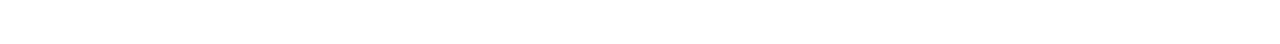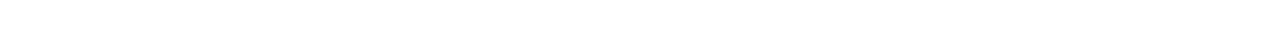# 15.1.3 Circular Orbits in Gravitational Fields

### Circular Orbits in Gravitational Fields

• Since most planets and satellites have a near circular orbit, the gravitational force FG between the sun or another planet provides the centripetal force needed to stay in an orbit
• Both the gravitational force and centripetal force are perpendicular to the direction of travel of the planet
• Consider a satellite with mass m orbiting Earth with mass M at a distance r from the centre travelling with linear speed v• This means that all satellites, whatever their mass, will travel at the same speed v in a particular orbit radius r
• Recall that since the direction of a planet orbiting in circular motion is constantly changing, it has centripetal acceleration

#### Kepler’s Third Law of Planetary Motion

• For the orbital time period T to travel the circumference of the orbit 2πr, the linear speed v can be written as• This is a result of the well-known equation, speed = distance / time
• Substituting the value of the linear speed v into the above equation:• The equation shows that the orbital period T is related to the radius r of the orbit. This is known as Kepler’s third law:

For planets or satellites in a circular orbit about the same central body, the square of the time period is proportional to the cube of the radius of the orbit

• Kepler’s third law can be summarised as:#### Maths Tip

• The ∝ symbol means ‘proportional to’
• Find out more about proportional relationships between two variables in the “proportional relationships ” section of the A Level Maths revision notes

#### Worked example: Circular orbits in gravitational fields

A binary star system constant of two stars orbiting about a fixed point B.

The star of mass M1 has a circular orbit of radius R1 and mass M2 has a radius of R2. Both have linear speed v and an angular speed ⍵ about B.State the following formula, in terms of G, M2, R1 and R2.

1. The angular speed ⍵ of M1
2. The time period T for each star in terms of angular speed

#### Exam Tip

Many of the calculations in the Gravitation questions depend on the equations for circular motion. Be sure to revisit these and understand how to use them!### Author: Katie

Katie has always been passionate about the sciences, and completed a degree in Astrophysics at Sheffield University. She decided that she wanted to inspire other young people, so moved to Bristol to complete a PGCE in Secondary Science. She particularly loves creating fun and absorbing materials to help students achieve their exam potential.
CloseClose